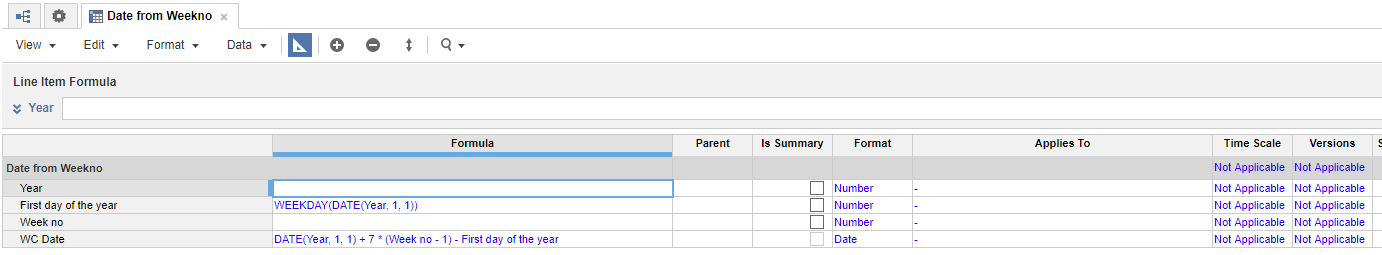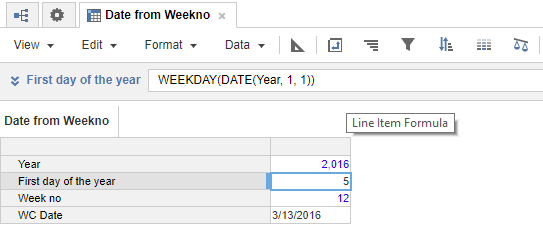English

## Use SUM or LOOKUP?

Determining whether to use SUM or LOOKUP depends on how the mapping table is set up between the Source List (where the original data resides) and the Target List (where the formula pulling the data resides).

A mapping table between the Source List and Target List can be set up in one of two places:

• in a module, using a list-formatted line item
• in a list, using a list-formatted property.

### General rule

If your mapping table contains the Target List and maps to a Source list-formatted line item or list-formatted property, then use LOOKUP in your formula. Setting your mapping table up this way says that each Target item can map to one and only one Source list item in the mapping table, so we are looking up that Source item value.

If your mapping table contains the Source list and maps to a Target list-formatted line item or list-formatted property, then use SUM in your formula. Setting your mapping table up this way says that each Target item can map to many Source items, so we are summing up all Source data that is to land in each Target list item. This is similar to SUMIF in Excel.

Questions to determine whether to use SUM or LOOKUP:

1. What's my Target list, where I want to write the formula and summarize or break out the data?
2. What's my Source list, where the data currently is?
3. How do I map my Target and Source lists?
4. What is the structure of this mapping table?

Once you have an answer for question #3, then refer to the general rule above.

## Permutations

For permutations where repetition is allowed, use POWER, with the number of items as x and the number of permutations as n.

## Convert a number to a Date

When you import data from a .csv file, the dates may be in a number format such as 20170630 (yyyymmdd) in a number-formatted line item. To convert this to a date format in Anaplan, you must use a combination of functions to convert the data to a date-formatted line item.

Use the LEFT and MID functions to extract sub-strings from the number format. Because LEFT only works on text data, you have to convert the number to text before extract, but the date format requires numerical data, so you need to convert the trimmed data back to a number afterwards.

DATE(VALUE(LEFT(TEXT(Number), 4)), VALUE(MID(TEXT(Number), 5, 2)), VALUE(RIGHT(TEXT(Number), 2)))

## Calculating Percentages

Percentages are calculated using line items. Create a line item that will hold your percentage result, formatted in Blueprint as a number. In the Format dialog, set the units to Percentage. The result of your calculation will be displayed as a percentage.

## CUMULATE

The CUMULATE function can be used with lists. You can use it across items of a named list, and the cumulate summing will follow the original order. You can use it to cumulate sums from the first time period across the entire timescale, and you can reset the cumulate amount with a Boolean. See CUMULATE for more.

## MOVINGSUM

Though the formula defaults to SUM, you can use all of the aggregate functions in Anaplan including AVERAGE, MIN, MAX, ANY, ALL, FIRST_NON_BLANK, LAST_NON_BLANK, and TEXTLIST. This formula requires the time dimension, because the values are always relative to the period that contains the result. See MOVINGSUM for more.

### Formula Syntax

MOVINGSUM(Item,From,To,Aggregate)

#### MOVING PRODUCT

There's no direct formula to calculate MOVING PRODUCT; you can create a line item that calculates the Natural Log (LN) of your value, and perform the MOVINGSUM on that line item. Then take the EXP of the MOVINGSUM value for MOVING PRODUCT.

Note: The LOG function does not work with zero or negative numbers. If you expect those numbers in your Value line item, use a line item to determine whether the value is negative and use the ABS function to return just the value. You can then perform a second MOVINGSUM to count the negative values in the line item in the range to determine whether the final figure should be a negative or positive value.

### Formula in this example

 Line Item Formula ABS(val) ABS(val) Evaluate val sign IF val < 1 THEN 1 ELSE 0 Movingsum of Evaluate val sign MOD(MOVINGSUM(Evaluate val sign, -2, 0), 2) Natural Log of ABS(val) LN('ABS(val)') MovingSum of Natural Log of ABS(val) MOVINGSUM('Natural Log of ABS(val)', -2, 0) Moving Product IF MOD(MOVINGSUM(Evaluate val sign, -2, 0), 2) = 1 THEN EXP('MovingSum of Natural Log of ABS(val)') * -1 ELSE EXP('MovingSum of Natural Log of ABS(val)')

## RANKCUMULATE

The RANKCUMULATE formula enables Anaplanners to cumulate against a list with ranking criteria. Being able to see the additive quantities of items gives you more flexibility.

This formula allows you to not only rank values in a list, but to cumulate them in a running aggregate as well. You can also include groupings to partition the rankings. This formula is useful for calculating total-to-date components, for example when calculating Sales Commissions.

Transactions attached to a sales rep can be aggregated by Sales Person (group) and based on the Date Sold (ranking) with the RANKCUMULATE formula. Once cumulated, you can compare against your goal and assess your payout rate.

### Formula Syntax

RANKCUMULATE(CumulationSource,Date Sold,Direction,Include,Sales Person)

## FINDITEM

This formula finds a valid item in a list or time period list by matching a text string to the list line items. It takes a list as the first parameter, and text as the second parameter, then matches the text to a list line item. You can use FINDITEM to search a list and return a matched list line item, if it belongs to the list. The key to this formula is to make sure that you assign codes to your lists, especially for numbered lists.

## Use SELECT and COLLECT with line items to calculate Variance

Set up the line items described below to derive actual and budget data from another module using the SELECT function. Having derived the actual and budget values, you can then build simple calculations for the variance and variance percent line items. The other advantage of using line items, rather than adding versions, is the ability to format each line item and use conditional formatting to highlight data.

 Line Item Formula Actual Module.Line Item[SELECT: VERSIONS.Actual] Budget Module.Line Item[SELECT: VERSIONS.Budget] Variance Budget - Actual Variance % Variance / Actual

You may encounter a problem where you already have line items in the module that you also want to use. To avoid this issue, create a line item subset and use the COLLECT function to pull data from line items in other modules. A line item subset allows you to group line items from different modules together to use as a single general list.

This procedure describes how to create a module that uses a line item subset and the COLLECT function to pull data from line items in other modules and use line items to calculate variance and variance percent.

1. Spend some time analyzing your model to understand which modules and line items require variance and variance percent calculations. This results in a much more effective use of your workspace.
2. Once you know the modules and line items you want to group together as a list, create a line item subset, and select those line items, across modules, for which you want to calculate Variance and Variance Percent.
3. Create a new module with these dimensions:
• Rows: <new line item subset>
• Columns: Line items (Data, Actual, Budget, Variance, Variance %)
4. In Blueprint, select the data line item.
5. In formula edit mode, enter COLLECT() and press Enter. Data for each item in the line item subset list will be populated with the data from the underlying line item.
6. Complete the calculations for the remaining line items, referring to the formula examples in the table above.
7. Format the line item data types, and add any conditional formatting.
8. Publish the module to a dashboard.

## Calculate the week start date from the week number

Set up three line items with Number format, and one with Date format, as shown below;

 Line item name Formula Format Year Number First Day of the year WEEKDAY(DATE(Year, 1, 1)) Number Week No Number WC Date DATE(Year, 1, 1) + 7 * (Week no - 1) - First day of the year Date

### Configuration shown in Blueprint### Results in the module viewDisclaimer

We update Anapedia content regularly to provide the most up-to-date instructions.